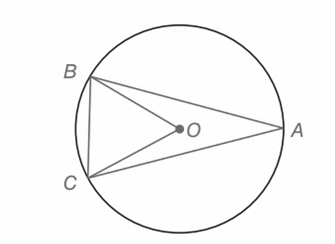Chapter 6.CT, Problem 3CTElementary Geometry For College St...

7th Edition
Alexander + 2 others
ISBN: 9781337614085

Solutions

Chapter
SectionElementary Geometry For College St...

7th Edition
Alexander + 2 others
ISBN: 9781337614085
Textbook Problem

a) If m ∠ B A C = 24 ° , then m B C ⌢ = _____________.b) If A B ⌢ ≅ A C ⌢ , then Δ A B C is a(n)___________ triangle.To determine

(a)

To find:

The measure of mBOC

Explanation

Given:

mBAC=24°

Approach:

1) In a circle the measure of an arc is twice the measure of the interior angle that intercepts the arc.

2) In a circle if two arcs are congruent, then the chord that intercepts the arcs are congruent.

Calculation:

Consider the given circle,

mBAC=24°.

BC is the arc that intercept the interior angle

To determine

(b)

To find:

The measure of mBAC

Still sussing out bartleby?

Check out a sample textbook solution.

See a sample solution

The Solution to Your Study Problems

Bartleby provides explanations to thousands of textbook problems written by our experts, many with advanced degrees!

Get Started

In Exercises 39-54, simplify the expression. (Assume that x, y, r, s, and t are positive.) 41. (x2y3) (x5y3)

Applied Calculus for the Managerial, Life, and Social Sciences: A Brief Approach

Differentiate. h(t)=6t+16t1

Calculus (MindTap Course List)

The equation of the tangent plane to for is:

Study Guide for Stewart's Multivariable Calculus, 8th